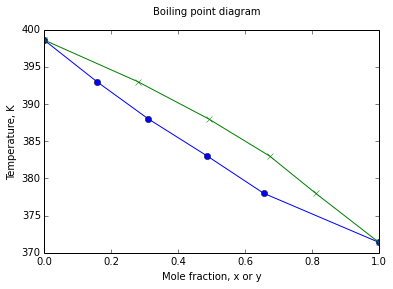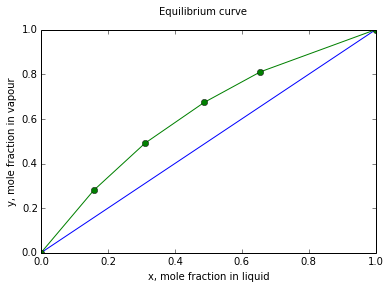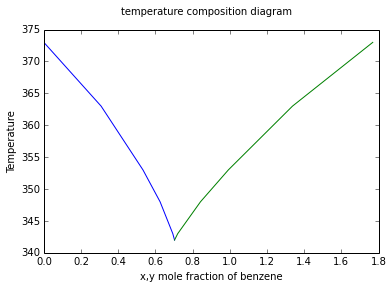# Chapter 7 : Solutions and Phase Behaviour¶

## Example 7.1 pageno : 155¶

In :
# variables
Pas = 71.2                      #kPa n-heptane
Pbs = 48.9                      #kPa toluene
P = 65.                         #kPa equilibrium

# Calculation
#P=(Pas-Pbs)*xa+Pbs,xa=mole fraction of n-heptane,liq. condition,therefore
xa = (P - Pbs)/(Pas - Pbs)
#ya = Pa / P , Vapour condition
ya = Pas * xa / P
P1 = xa * 100
P2 = ya * 100

# Result
print "Percentage of hepatne in liquid = %.1f %%"%P1
print "Percentage of hepatne in vapour = %.1f %%"%P2

Percentage of hepatne in liquid = 72.2 %
Percentage of hepatne in vapour = 79.1 %


## Example 7.2 pageno : 155¶

In :
# variables
P1 = 100.               #kPa ( Vapour pressure of liq A )
P2 = 60.                #kPa ( Vapour pressure of liq B )
T = 320.                #K

# Calculation
#Pa = xa * P1 = 100 * xa
#Pa = xb * P2 = 60 * xb
#P = xa * P1 + ( 1 - xa )* P2
# = 100xa + ( 1 - xa )* 60
# = 60 + 40*xa
#ya = Pa / P
#0.5 = 100*xa / ( 60 + 40 * xa)
xa = 60 * 0.5 / (100 - 20)
Per1 = xa * 100

# Result
print "(a)Percentage of A in liquid = ",Per1,"%"
Ptotal = 60 + 40 * xa
print "(b)Total pressure of the vapour = ",Ptotal,"kPa"

(a)Percentage of A in liquid =  37.5 %
(b)Total pressure of the vapour =  75.0 kPa


## Example 7.3 pageno : 156¶

In :
# variables
xa = 0.25          # liquid mixture
xb = 0.30

# Calculation
xc = 1 - xa - xb
Ptotal = 200.                   #kPa
Pcs = 50.                       #kPa(Vapour pressure of c)
Pc = xc * Pcs                   #(partial pressure of c)
yc = Pc / Ptotal
yb = 0.5
ya = 1 - yb - yc
per1 = ya * 100

# Result
print "Percentage of A in vapour = ",per1,"%"

Percentage of A in vapour =  38.75 %


## Example 7.4 pageno : 156¶

In :
# variables
P = 101.3                       #kPa flash vaporization
Pbs = 54.21                     #kPa pressure
Pas = 136.09                    #kPa temperature
xf = 0.65                       # liquid mixture

# Calculation
xw = (P - Pbs)/(Pas - Pbs)
yd = xw * Pas / P
# f = ( xf - xw ) / ( yd - xw )
f = ( xf - xw ) / ( yd - xw )
per1 = f * 100

# Result
print "mole percent of the feed that is vapourised = %.1f %%"%per1

mole percent of the feed that is vapourised = 37.9 %


## Example 7.5 pageno : 157¶

In :
%matplotlib inline

from matplotlib.pyplot import *
from numpy import zeros

# variables
T = [371.4, 378 ,383 ,388, 393, 398.6]
Pas = [101.3, 125.3, 140 ,160, 179.9, 205.3]
Pbs = [44.4, 55.6, 64.5, 74.8, 86.6, 101.3]
Ptotal = 101.3                      #kPa

# Calculation
x = zeros(6)
y = zeros(6)
for i in range(6):
x[i] = (Ptotal - Pbs[i])/(Pas[i] - Pbs[i])
y[i] = x[i] * Pas[i] / Ptotal

# Result
#subplot(2,1,1)
plot(x,T,'-o')
plot(y,T,'-x')
suptitle("Boiling point diagram")
xlabel("Mole fraction, x or y")
ylabel("Temperature, K")
show()

# part 2

# variables
T = [371.4, 378, 383, 388, 393, 398.6]
Pas = [101.3, 125.3, 140, 160, 179.9, 205.3]
Pbs = [44.4, 55.6, 64.5, 74.8, 86.6, 101.3]
Ptotal = 101.3                                      #kPa
x = zeros(6)
y = zeros(6)

# Calculation
for i in range(6):
x[i] = (Ptotal - Pbs[i])/(Pas[i] - Pbs[i])
y[i] = x[i] * Pas[i] / Ptotal

w = x
# Result
#subplot(2,1,2)
plot(x,w)
plot(x,y,'-o')
suptitle("Equilibrium curve")
xlabel("x, mole fraction in liquid")
ylabel("y, mole fraction in vapour")
show()

Populating the interactive namespace from numpy and matplotlib## Example 7.6 page no : 158¶

In :
import math

# variables
p = 100                   # pressure Kpa
# from table
n_heptaneA = 13.8587
n_hexaneA = 13.8216
n_heptaneB = 2911.32
n_hexaneB = 2697.55
n_heptaneC = 56.51
n_hexaneC = 48.78
xA = .25

# Calculations and Results

# for T = 360   Initial guess
T1 = 360
lnPaS = n_hexaneA - (n_hexaneB/(T1- n_hexaneC))
PaS = round(math.e**lnPaS,2)
lnPbS = n_heptaneA - (n_heptaneB/(T1- n_heptaneC))
PbS = round(math.e**lnPbS,2)
P = xA*PaS + (1 - xA)*PbS

print "P = %.1f kPa" %P

# Since total pressure < 100 Kpa, equilibrium temperature is not equal to 360K.
# for T = 365 - Assume
T1 = 365
lnPaS = n_hexaneA - (n_hexaneB/(T1- n_hexaneC))
PaS = round(math.e**lnPaS,2)
lnPbS = n_heptaneA - (n_heptaneB/(T1- n_heptaneC))
PbS = round(math.e**lnPbS,2)
P = xA*PaS + (1 - xA)*PbS

print "P = %.2f kPa"%P

# As the pressure > 100 kPa, the temperature lies between 360 and 365 K.
# for T = 361.125 -- assumption
T1 = 361.125
lnPaS = n_hexaneA - (n_hexaneB/(T1- n_hexaneC))
PaS = round(math.e**lnPaS,2)
lnPbS = n_heptaneA - (n_heptaneB/(T1- n_heptaneC))
PbS = round(math.e**lnPbS,2)
P = xA*PaS + (1 - xA)*PbS

print "P = %.f kPa"%P
print "Therefore, the bubble point temperature = %.3f K"%T1   # last assumed for T1

# Part b
Pa = xA*PaS
Ya = Pa/P

print "Partial pressure of n-Hexane in vapour at the bubble point is = %.2f kPa"%Pa
print "Mole fraction of hexane in the vapour is Ya = %.4f"%Ya
print "The vapour contains %.2f %% hexane and %.2f %% heptane."%(Ya*100,(1-Ya)*100)

P = 96.7 kPa
P = 112.04 kPa
P = 100 kPa
Therefore, the bubble point temperature = 361.125 K
Partial pressure of n-Hexane in vapour at the bubble point is = 44.65 kPa
Mole fraction of hexane in the vapour is Ya = 0.4465
The vapour contains 44.65 % hexane and 55.35 % heptane.


## Example 7.7 page no : 159¶

In :
import math

# Variables
#lnPas = 14.5463 - 2940.46/(T - 35.93)
#lnPbs = 14.2724 - 2945.47 / (T - 49.15)
#xa = (P - Pbs)/(Pas - Pbs)
#Ya = Pas * (P - Pbs)/(P * (Pas - Pbs))
Ya = 0.4                            # vapour phase
P = 65.                             #kPa
#various temperature value are assumed and tried till LHS = RHS, we get
T = 334.15                          #K

# Calculation
Pas = math.exp(14.5463 - 2940.46/(T - 35.93))
Pbs = math.exp(14.2724 - 2945.47 / (T - 49.15))
xa = (P - Pbs)/(Pas - Pbs)

# Result
print "(a)The Dew point temperature at 65 kPa = ",T,"K"
print "    Concentration of the first drop of liquid = %.2f"%xa
T1 = 327.                           #K
Pas1 = math.exp(14.5463 - 2940.46/(T1 - 35.93))
Pbs1 = math.exp(14.2724 - 2945.47 / (T1 - 49.15))
xa1 = Ya * Pbs1 / (Pas1 - Ya*(Pas1 - Pbs1))
P1 = xa1 * Pas1 / Ya
print "(b)The dew point pressure at 327 K = %.2f kPa"%P1
print "    Concentration at 327K = %.4f"%xa1

(a)The Dew point temperature at 65 kPa =  334.15 K
Concentration of the first drop of liquid = 0.24
(b)The dew point pressure at 327 K = 50.09 kPa
Concentration at 327K = 0.2354


## Example 7.8 pageno : 163¶

In :
# variables
MW = 44.032                # mole fraction of acetaldehyde
Mwater = 18.016
x = 2.                      #%    acetaldehyde weight
Pa = 41.4                   #kPa   solution

# Calculation
Mfr = (x/MW)/(x/MW + (100-x)/Mwater)
#henry's law gives Pa = Ha * xa
Ha = Pa / Mfr
Molality = 0.1
Mfr1 = Molality / (1000/Mwater + Molality)
Pa1 = Ha * Mfr1

# Result
print "Partial Pressure = %.f kPa"%Pa1

Partial Pressure = 9 kPa


## Example 7.9 pageno : 177¶

In :
import math

# Variables
#1 - pentane, 2 - hexane, 3 - heptane

x1 = 0.6
x2 = 0.25
x3 = 0.15
A1 = 13.8183
A2 = 13.8216
A3 = 13.8587
B1 = 2477.07
B2 = 2697.55
B3 = 2911.32
C1 = 39.94
C2 = 48.78
C3 = 56.51
#As raoults law is applicable, Ki = yi/xi = Pis/P
#yi = xi*Pis/P
#ln P = A- B/(T-C)
#Assuming,
P = 400.                #kPa
T = 369.75              #K

# Calculation
Pas1 = math.exp(A1 - B1 / (T - C1))
Pas2 = math.exp(A2 - B2 / (T - C2))
Pas3 = math.exp(A3 - B3 / (T - C3))
Yi = (x1*Pas1 + x2*Pas2 + x3*Pas3)/P
print "(a)bubble point temperature of the mixture = ",T,"K"
y1 = x1*Pas1/P
y2 = x2*Pas2/P
y3 = x3*Pas3/P

# Result
print "(b)composition of n-pentane in vapour = %.2f %%"%(y1*100)
print "composition of n-hexane in vapour = %.2f %%"%(y2*100)
print "composition of n-heptane in vapour = %.2f %%"%(y3*100)
T1 = 300.               #K
Ps1 = math.exp(A1 - B1 / (T1 - C1))
Ps2 = math.exp(A2 - B2 / (T1 - C2))
Ps3 = math.exp(A3 - B3 / (T1 - C3))
P1 = x1*Ps1 + x2*Ps2 + x3*Ps3
print "(c)Bubble point pressure = %.1f kPa"%P1

(a)bubble point temperature of the mixture =  369.75 K
(b)composition of n-pentane in vapour = 82.32 %
composition of n-hexane in vapour = 14.08 %
composition of n-heptane in vapour = 3.60 %
(c)Bubble point pressure = 50.4 kPa


## Example 7.10 pageno : 178¶

In :
import math

# Variables
#1 - pentane, 2 - hexane, 3 - heptane

y1 = 0.6
y2 = 0.25
y3 = 0.15
A1 = 13.8183
A2 = 13.8216
A3 = 13.8587
B1 = 2477.07
B2 = 2697.55
B3 = 2911.32
C1 = 39.94
C2 = 48.78
C3 = 56.51
P = 400.                    #kPa
T = 300.                    #K
#As raoults law is applicable, Ki = yi/xi = Pis/P
#xi = yi*P/Pis
#ln P = A- B/(T-C)
#Assuming,
T1 = 385.94                 #K

# Calculation
Pas1 = math.exp(A1 - B1 / (T1 - C1))
Pas2 = math.exp(A2 - B2 / (T1 - C2))
Pas3 = math.exp(A3 - B3 / (T1 - C3))

# Result
print "(a)Dew point temperature of the mixture = ",T1,"K"
Ps1 = math.exp(A1 - B1 / (T - C1))
Ps2 = math.exp(A2 - B2 / (T - C2))
Ps3 = math.exp(A3 - B3 / (T - C3))
P1 = 1/(y1/Ps1 + y2/Ps2 + y3/Ps3)
print "(b)Dew point pressure = %.2f kPa"%P1

(a)Dew point temperature of the mixture =  385.94 K
(b)Dew point pressure = 23.79 kPa


## Example 7.11 page no :180¶

In :
# variables
#1 - methanol, 2 - ethanol, 3 - propanol
x1 = 0.45
x2 = 0.3
x3 = 1 - (x1 + x2)
P = 101.3                   #kPa
# by drawing the temperature vs vapour pressure graph and interpolation,assuming,
T = 344.6                   #K
Ps1 = 137.3
Ps2 = 76.2
Ps3 = 65.4

# Calculation
y1 = x1 * Ps1 / P
y2 = x2 * Ps2 / P
y3 = x3 * Ps3 / P

# Result
print "(a)Bubble point temperature = ",T,"K"
print "Composition of methanol in vapour = %.f %%"%(y1*100)
print "Composition of ethanol in vapour = %.1f%%"%(y2*100)
print "Composition of propanol in vapour = %.1f%%"%(y3*100)
#again, for xi = 1
T1 = 347.5                  #K
P1 = 153.28
P2 = 85.25
P3 = 73.31
xa = x1 * P / P1
xb = x2 * P / P2
xc = x3 * P / P3
print "(b)Dew point temperature = ",T1,"K"
print "Composition of methanol in liquid = %.1f %%"%(xa*100)
print "Composition of ethanol in liquid = %.1f %%"%(xb*100)
print "Composition of propanol in liquid = %.1f %%"%(xc*100)

(a)Bubble point temperature =  344.6 K
Composition of methanol in vapour = 61 %
Composition of ethanol in vapour = 22.6%
Composition of propanol in vapour = 16.1%
(b)Dew point temperature =  347.5 K
Composition of methanol in liquid = 29.7 %
Composition of ethanol in liquid = 35.6 %
Composition of propanol in liquid = 34.5 %


## Example 7.12 pageno : 181¶

In :
# variables
xp = 0.25                           # propane
xnb = 0.4                           # n-butane
xnp = 0.35                          # n-pentane
P = 1447.14                         #kPa
#assuming temperatures 355.4 K and 366.5 K , corresponding Ki
# values are found from nomograph and total Ki value are 0.928 and 1.075 resp, thus bubble point temperature lies between, using interpolation bubble point temperature is found to be,
Tb = 361.                           #K
print "(a) The buuble point temperature = ",Tb,"K"

# Calculation
#At 361,
Kip = 2.12
Kinb = 0.85
Kinp = 0.37
xp1 = Kip * xp
xnb1 = Kinb * xnb
xnp1 = Kinp * xnp

# Result
print "concentration of propane at bubble point = ",xp1
print "concentration of n-butane at bubble point = ",xnb1
print "concentration of n-pentane at bubble point = %.3f"%xnp1
#At dew point Yi/Ki = 1, at 377.6K this is 1.1598 and at 388.8K
# it is 0.9677, by interpolation dew point is found to be
Td = 387.                           #K
Kip1 = 2.85
Kinb1 = 1.25
Kinp1 = 0.59
yp1 = xp/Kip1
ynb1 = xnb/Kinb1
ynp1 = xnp/Kinp1
print "(b) The dew point temperature = ",Td,"K"
print "concentration of propane at dew point = %.4f"%yp1
print "concentration of n-butane at dew point = %.4f"%ynb1
print "concentration of n-pentane at dew point = %.4f"%ynp1

#summation zi / (1 + L/VKi)= 0.45, using trial and error, we find
T = 374.6                           #K
L = 0.55
V = 0.45
Kip2 = 2.5
Kinb2 = 1.08
Kinp2 = 0.48
t = (xp/(1+L/(V*Kip2)))+(xnb/(1+L/(V*Kinb2))) + (xnp/(1+L/(V*Kinp2)))
yp2 = (xp/(1+L/(V*Kip2)))/t
ynb2 = (xnb/(1+L/(V*Kinb2)))/t
ynp2 = (xnp/(1+L/(V*Kinp2)))/t
xp2 = (xp - V * yp2)/L
xnb2 = (xnb - V * ynb2)/L
xnp2 = (xnp - V * ynp2)/L
print "(c)Temperature of the mixture = ",T,"K"
print "vapour phase concentration of propane = %.4f"%yp2
print "vapour phase concentration of n-butane = %.4f"%ynb2
print "vapour phase concentration of n-pentane = %.4f"%ynp2
print "liquid phase concentration of propane = %.4f"%xp2
print "liquid phase concentration of n-butane = %.4f"%xnb2
print "liquid phase concentration of n-pentane = %.4f"%xnp2

(a) The buuble point temperature =  361.0 K
concentration of propane at bubble point =  0.53
concentration of n-butane at bubble point =  0.34
concentration of n-pentane at bubble point = 0.130
(b) The dew point temperature =  387.0 K
concentration of propane at dew point = 0.0877
concentration of n-butane at dew point = 0.3200
concentration of n-pentane at dew point = 0.5932
(c)Temperature of the mixture =  374.6 K
vapour phase concentration of propane = 0.3696
vapour phase concentration of n-butane = 0.4131
vapour phase concentration of n-pentane = 0.2173
liquid phase concentration of propane = 0.1521
liquid phase concentration of n-butane = 0.3893
liquid phase concentration of n-pentane = 0.4586


## Example 7.13 pageno : 185¶

In :
# variables
P = 93.30               #kPa   pressure
T1 = 353.               #K     temperature
T2 = 373.               #K     temperature
Pwater1 = 47.98         #kPa   pressure of water
Pwater2 = 101.3         #kPa
Pliq1 = 2.67            #kPa   pressure of liquid
Pliq2 = 5.33            #kPa

# Calculation
T = T1 + (T2 - T1)*(P - (Pwater1 + Pliq1))/(Pwater2 + Pliq2 - (Pwater1 + Pliq1))

# Result
print "(a)The equilibrium temperature = %.1f K"%T
Pwater = 88.50
y = Pwater * 100 /P
print "(b)Water vapour in vapour mixture = %.2f %%"%y

(a)The equilibrium temperature = 368.2 K
(b)Water vapour in vapour mixture = 94.86 %


## Example 7.14 pageno : 185¶

In :
%matplotlib inline

from matplotlib.pyplot import *
from numpy import *

# variables
#the three phase temperature is first find out, which comes to be 342K, the
#  corresponding Ps1 = 71.18, Ps2 = 30.12
T = [342, 343, 348, 353, 363, 373]
Ps2 = [30.12, 31.06, 37.99, 47.32, 70.11, 101.3]
Ps1 = [71.18, 72.91, 85.31, 100.5, 135.42, 179.14]
P = 101.3                   #kPa

y1 = zeros(6)
y2 = zeros(6)

# Calculation
for i in range(6):
y1[i] = 1 - (Ps1[i])/P

for i in range(6):
y2[i] = 1 - (Ps2[i])/P

# Result
plot(y2,T)
plot(1-y1,T)
suptitle("temperature composition diagram")
xlabel("x,y mole fraction of benzene")
ylabel("Temperature")
show()

Populating the interactive namespace from numpy and matplotlib

WARNING: pylab import has clobbered these variables: ['draw_if_interactive']
%pylab --no-import-all prevents importing * from pylab and numpy## Example 7.15 pageno : 188¶

In :
import math

# Variables
T = 379.2               #K   solution
P = 101.3               #kPa
Ps = 70.                #kPa solution
Molality = 5

# Calculation
Pws = math.exp(16.26205 - 3799.887/(T - 46.854))
k = P / Pws
Pws1 = Ps / k
T1 = 3799.887 / (16.26205 - math.log( Pws1)) + 46.854

# Result
print "Boiling point of the solution = %.1f K"%T1

Boiling point of the solution = 368.8 K